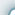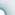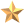Coming Soon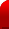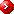College Mathematics 1 – (XI Grade)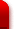PreliminariesArithmetic and Geometric ProgressionsRemainder and Factor Theorems Sets Indices and Logarithms Arithmetic Progressions Arithmetic Series Geometric Progressions Geometric Series Compound Interest Infinite Geometric Series The Summation Notation Polynomials Algebraic Operations on Polynomial The Remainder Theorem The Factor TheoremMathematical Induction Permutations and Combinations Partial Fractions and the Binomial Theorem Introduction The Principle Introduction Permutations Two Basic Principles More on Permutations Combinations Partial Fractions The Binomial Theorem The Binomial Series Quadratic Equations Inequalities Complex Numbers Quadratic Expressions Quadratic Expressions Range of Values of ax2 + bx + c Basic Concepts and Results Inequalities on One Variable Inequalities on Two Variables The Complex Number System The Argand Diagram Trigonometric Form of a Complex Number Multiplication, Division and De Moivre's Theorem Geometry in the Argand Diagram Relations and Functions Trigonometry Vectors Relations Functions Some Basic Algebraic Functions Simple Transformations on Graphs One-one and Onto Functions Inverse Functions Composite Functions Radian and Degree Measures of an Angle The Trigonometrical Functions The Graphs of Trigonometrical Functions The Inverse Trigonometrical Functions Fundamental Identities General Solutions of Trigonometrical Equations Problems in Three Dimensional Space Introduction Geometrical Representation of Vectors Fundamental Results and the Ratio Theorem Position Vectors Two Dimensional Vectors in Cartesian Form Three Dimensional Vectors in Cartesian Form Vector Equations of Lines and Planes Scalar Product of Two Vectors Projections Equation of a Circle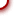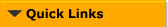cybeRShala Difference Lesson Illustration How It Works Compare Us Request Info Placement Test Tell a friend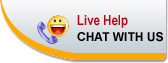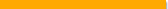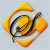Home | FAQ | Tell a Friend | Contact Us | Compare Us | Homeschool Resources | Sitemap cybershala.com Inc. All Rights reserved | Terms of service | Privacy policy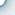s